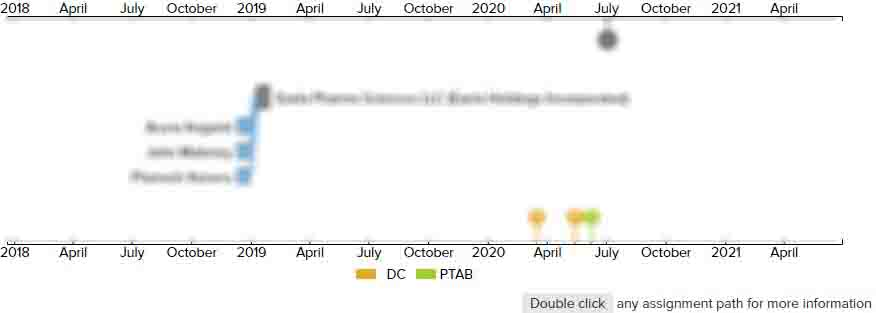# Mathematical teaching apparatus and method

• US 6,206,701 B1
• Filed: 07/13/1999
• Issued: 03/27/2001
• Est. Priority Date: 11/19/1996
• Status: Expired due to Term
##### First Claim
Patent Images

1. A method for teaching mathematical division, said method comprising:

• providing several physical elements, each element containing ten lesser physical elements;

providing instructions to divide the elements into a number of piles;

distributing the elements, one in each pile, as long as each pile contains an equal number of elements;

when any elements remain that cannot be distributed equally to each pile, opening those elements to obtain the contained ten lesser elements;

then, distributing the lesser elements equally to each pile until there remains only a remaining number of lesser elements that cannot be distributed equally to each pile;

when further division is desired, opening each of the remaining number of lesser elements to obtain ten even lesser elements from each one, and distributing the contents of the lesser elements evenly into the piles until only a remainder number of such even lesser elements cannot be evenly divided into the piles;

wherein each of the remainder number can be opened to obtain ten even smaller elements and the opening and distributing steps can be repeated until the desired degree of division is obtained; and

identifying any remaining number of whole elements as the remainder.

• ##### 2 Assignments
Timeline View
Assignment View×
×